# NCERT Solutions For Class 11 Physics Thermal Properties of Matter## myCBSEguide App

CBSE, NCERT, JEE Main, NEET-UG, NDA, Exam Papers, Question Bank, NCERT Solutions, Exemplars, Revision Notes, Free Videos, MCQ Tests & more.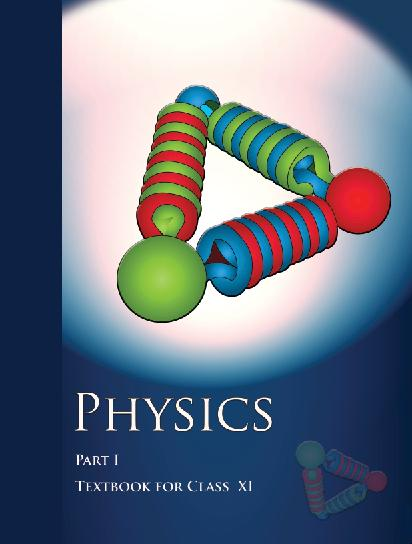## NCERT Class 11 Physics Chapter wise Solutions

• 1 – Physical World
• 2 – Units and Measurements
• 3 – Motion in a Straight line
• 4 – Motion in a Plane
• 5 – Laws of Motion
• 6 – Work, Energy and Power
• 7 – System of Particles and Rotational motion
• 8 – Gravitation
• 9 – Mechanical Properties of Solids
• 10 – Mechanical properties of fluids
• 11 – Thermal Properties of matter
• 12 – Thermodynamics
• 13 – Kinetic Theory
• 14 – Oscillations
• 15 – Waves

## CHAPTER 11 THERMAL PROPERTIES OF MATTER

• 11.1 Introduction
• 11.2 Temperature and heat
• 11.3 Measurement of temperature
• 11.4 Ideal-gas equation and absolute temperature
• 11.5 Thermal expansion
• 11.6 Specific heat capacity
• 11.7 Calorimetry
• 11.8 Change of state
• 11.9 Heat transfer
• 11.10 Newton’s law of cooling

## NCERT Solutions For Class 11 Physics Thermal Properties of Matter

1. The triple points of neon and carbon dioxide are 24.57 K and 216.55 K respectively. Express these temperatures on the Celsius and Fahrenheit scales.

2. Two absolute scales A and B have triple points of water defined to be 200 A and 350 B. What is the relation between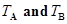?

3. The electrical resistance in ohms of a certain thermometer varies with temperature according to the approximate law: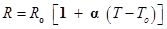The resistance is 101.6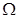at the triple-point of water 273.16 K, and 165.5at the normal melting point of lead (600.5 K). What is the temperature when the resistance is 123.4?

(a) The triple-point of water is a standard fixed point in modern thermometry. Why? What is wrong in taking the melting point of ice and the boiling point of water as standard fixed points (as was originally done in the Celsius scale)?

(b) There were two fixed points in the original Celsius scale as mentioned above which were assigned the number 0 °C and 100 °C respectively. On the absolute scale, one of the fixed points is the triple-point of water, which on the Kelvin absolute scale is assigned the number 273.16 K. What is the other fixed point on this (Kelvin) scale?

(c) The absolute temperature (Kelvin scale) T is related to the temperature tc on the Celsius scale by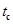= T – 273.15

Why do we have 273.15 in this relation, and not 273.16?

(d) What is the temperature of the triple-point of water on an absolute scale whose unit interval size is equal to that of the Fahrenheit scale?

5. Two ideal gas thermometers A and Buse oxygen and hydrogen respectively. The following observations are made: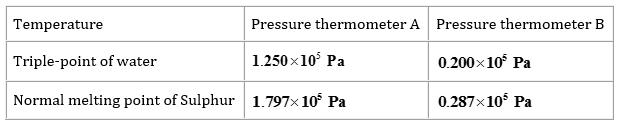(a) What is the absolute temperature of normal melting point of sulphur as read by thermometers A and B?

(b) What do you think is the reason behind the slight difference in answers of thermometers A and B? (The thermometers are not faulty). What further procedure is needed in the experiment to reduce the discrepancy between the two readings?

6. A steel tape 1m long is correctly calibrated for a temperature of 27.0 °C. The length of a steel rod measured by this tape is found to be 63.0 cm on a hot day when the temperature is 45.0 °C. What is the actual length of the steel rod on that day? What is the length of the same steel rod on a day when the temperature is 27.0 °C? Coefficient of linear expansion of steel =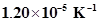.

7. A large steel wheel is to be fitted on to a shaft of the same material. At 27 °C, the outer diameter of the shaft is 8.70 cm and the diameter of the central hole in the wheel is 8.69 cm. The shaft is cooled using ‘dry ice’. At what temperature of the shaft does the wheel slip on the shaft? Assume coefficient of linear expansion of the steel to be constant over the required temperature range: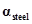=.

8. A hole is drilled in a copper sheet. The diameter of the hole is 4.24 cm at 27.0 °C. What is the change in the diameter of the hole when the sheet is heated to 227 °C? Coefficient of linear expansion of copper =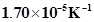.

9. A brass wire 1.8 m long at 27 °C is held taut with little tension between two rigid supports. If the wire is cooled to a temperature of -39 °C, what is the tension developed in the wire, if its diameter is 2.0 mm? Co-efficient of linear expansion of brass =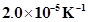; Young’s modulus of brass =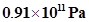.

10. A brass rod of length 50 cm and diameter 3.0 mm is joined to a steel rod of the same length and diameter. What is the change in length of the combined rod at 250 °C, if the original lengths are at 40.0 °C? Is there a ‘thermal stress’ developed at the junction? The ends of the rod are free to expand (Co-efficient of linear expansion of brass =, steel =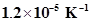).

11. The coefficient of volume expansion of glycerin is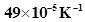. What is the fractional change in its density for a 30 °C rise in temperature?

12. A 10 kW drilling machine is used to drill a bore in a small aluminium block of mass 8.0 kg. How much is the rise in temperature of the block in 2.5 minutes, assuming 50% of power is used up in heating the machine itself or lost to the surroundings. Specific heat of aluminium = 0.91 J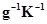.

13. A copper block of mass 2.5 kg is heated in a furnace to a temperature of 500 °C and then placed on a large ice block. What is the maximum amount of ice that can melt? (Specific heat of copper = 0.39 J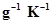; heat of fusion of water = 335 J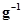).

14. In an experiment on the specific heat of a metal, a 0.20 kg block of the metal at 150 °C is dropped in a copper calorimeter (of water equivalent 0.025 kg) containing 150 cm3 of water at 27 °C. The final temperature is 40 °C. Compute the specific heat of the metal. If heat losses to the surroundings are not negligible, is your answer greater or smaller than the actual value for specific heat of the metal?

15. Given below are observations on molar specific heats at room temperature of some common gases.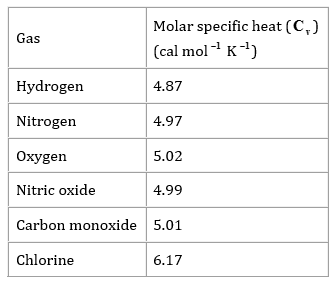The measured molar specific heats of these gases are markedly different from those for monatomic gases. Typically, molar specific heat of a monatomic gas is 2.92 cal/mol K. Explain this difference. What can you infer from the somewhat larger (than the rest) value for chlorine?

16. Answer the following questions based on the PT phase diagram of carbon dioxide:

(a) At what temperature and pressure can the solid, liquid and vapour phases of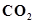co-exist in equilibrium?

(b) What is the effect of decrease of pressure on the fusion and boiling point of?

(c) What are the critical temperature and pressure for? What is their significance?

(d) Issolid, liquid or gas at (a) -70 °C under 1 atm, (b) -60 °C under 10 atm, (c) 15 °C under 56 atm?

17. Answer the following questions based on the P-T phase diagram of:

(a)at 1 atm pressure and temperature – 60 °C is compressed isothermally. Does it go through a liquid phase?

(b) What happens whenat 4 atm pressure is cooled from room temperature at constant pressure?

(c) Describe qualitatively the changes in a given mass of solidat 10 atm pressure and temperature -65 °C as it is heated up to room temperature at constant pressure.

(d)is heated to a temperature 70 °C and compressed isothermally. What changes in its properties do you expect to observe?

18. A child running a temperature of 101°F is given an antipyrin (i.e. a medicine that lowers fever) which causes an increase in the rate of evaporation of sweat from his body. If the fever is brought down to 98 °F in 20 min, what is the average rate of extra evaporation caused, by the drug? Assume the evaporation mechanism to be the only way by which heat is lost. The mass of the child is 30 kg. The specific heat of human body is approximately the same as that of water, and latent heat of evaporation of water at that temperature is about 580 cal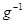.

19. A ‘thermacole’ icebox is a cheap and efficient method for storing small quantities of cooked food in summer in particular. A cubical icebox of side 30 cm has a thickness of 5.0 cm. If 4.0 kg of ice is put in the box, estimate the amount of ice remaining after 6 h. The outside temperature is 45 °C, and co-efficient of thermal conductivity of thermacole is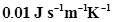. [Heat of fusion of water =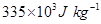]

20. A brass boiler has a base area of 0.15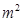and thickness 1.0 cm. It boils water at the rate of 6.0 kg/min when placed on a gas stove. Estimate the temperature of the part of the flame in contact with the boiler. Thermal conductivity of brass =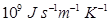; Heat of vaporisation of water =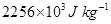.

21. Explain why:

(a) a body with large reflectivity is a poor emitter

(b) a brass tumbler feels much colder than a wooden tray on a chilly day

(c) an optical pyrometer (for measuring high temperatures) calibrated for an ideal black body radiation gives too low a value for the temperature of a red hot iron piece in the open, but gives a correct value for the temperature when the same piece is in the furnace

(d) the earth without its atmosphere would be inhospitably cold

(e) heating systems based on circulation of steam are more efficient in warming a building than those based on circulation of hot water

22. A body cools from 80 °C to 50 °C in 5 minutes. Calculate the time it takes to cool from 60 °C to 30 °C. The temperature of the surroundings is 20 °C.

## NCERT Solutions for Class 11 Physics

NCERT Solutions Class 11 Physics PDF (Download) Free from myCBSEguide app and myCBSEguide website. Ncert solution class 11 physics includes text book solutions from both part 1 and part 2. NCERT Solutions for CBSE Class 11 Physics have total 15 chapters. 11 Physics NCERT Solutions in PDF for free Download on our website. Ncert physics class 11 solutions PDF and physics ncert class 11 PDF solutions with latest modifications and as per the latest CBSE syllabus are only available in myCBSEguide

## CBSE app for Class 11

To download NCERT Solutions for class 11 Physics, Chemistry, Biology, History, Political Science, Economics, Geography, Computer Science, Home Science, Accountancy, Business Studies and Home Science; do check myCBSEguide app or website. myCBSEguide provides sample papers with solution, test papers for chapter-wise practice, NCERT solutions, NCERT Exemplar solutions, quick revision notes for ready reference, CBSE guess papers and CBSE important question papers. Sample Paper all are made available through the best app for CBSE students and myCBSEguide website